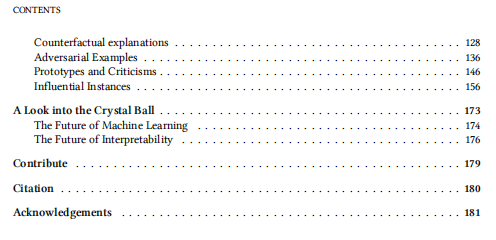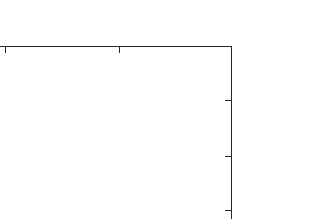### VIP内容• 前言
• 第一章 引言
• 第二章 解释性
• 第三章 数据集
• 第四章 解释模型
• 第五章 模型不可知论方法
• 第六章 基于实例的解释
• 第七章 神经网络解释
• 第八章 水晶球
• 第九章 贡献
• 第十章 引用本书0587

### 热门内容082

### 最新内容Nearly all statistical inference methods were developed for the regime where the number \$N\$ of data samples is much larger than the data dimension \$p\$. Inference protocols such as maximum likelihood (ML) or maximum a posteriori probability (MAP) are unreliable if \$p=O(N)\$, due to overfitting. This limitation has for many disciplines with increasingly high-dimensional data become a serious bottleneck. We recently showed that in Cox regression for time-to-event data the overfitting errors are not just noise but take mostly the form of a bias, and how with the replica method from statistical physics once can model and predict this bias and the noise statistics. Here we extend our approach to arbitrary generalized linear regression models (GLM), with possibly correlated covariates. We analyse overfitting in ML/MAP inference without having to specify data types or regression models, relying only on the GLM form, and derive generic order parameter equations for the case of \$L2\$ priors. Second, we derive the probabilistic relationship between true and inferred regression coefficients in GLMs, and show that, for the relevant hyperparameter scaling and correlated covariates, the \$L2\$ regularization causes a predictable direction change of the coefficient vector. Our results, illustrated by application to linear, logistic, and Cox regression, enable one to correct ML and MAP inferences in GLMs systematically for overfitting bias, and thus extend their applicability into the hitherto forbidden regime \$p=O(N)\$.

•000下载预览

### 最新论文Nearly all statistical inference methods were developed for the regime where the number \$N\$ of data samples is much larger than the data dimension \$p\$. Inference protocols such as maximum likelihood (ML) or maximum a posteriori probability (MAP) are unreliable if \$p=O(N)\$, due to overfitting. This limitation has for many disciplines with increasingly high-dimensional data become a serious bottleneck. We recently showed that in Cox regression for time-to-event data the overfitting errors are not just noise but take mostly the form of a bias, and how with the replica method from statistical physics once can model and predict this bias and the noise statistics. Here we extend our approach to arbitrary generalized linear regression models (GLM), with possibly correlated covariates. We analyse overfitting in ML/MAP inference without having to specify data types or regression models, relying only on the GLM form, and derive generic order parameter equations for the case of \$L2\$ priors. Second, we derive the probabilistic relationship between true and inferred regression coefficients in GLMs, and show that, for the relevant hyperparameter scaling and correlated covariates, the \$L2\$ regularization causes a predictable direction change of the coefficient vector. Our results, illustrated by application to linear, logistic, and Cox regression, enable one to correct ML and MAP inferences in GLMs systematically for overfitting bias, and thus extend their applicability into the hitherto forbidden regime \$p=O(N)\$.

•000下载预览
Top